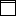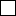(also use these if stuck in another frame)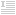Introduction |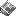News/Updates |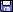Files |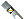Measurement |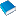Terminology/Script Commands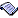RenderWare Scripting (RWX) |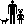Avatars |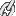Links |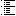Site Index |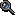SearchMeasurement

Units |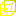Scale | Object Manipulation

To effectively create 3D objects, you should know the measurements of the environment in which they will be used.

Units

Measurement in RenderWare and Active Worlds is based off the metric system of units, specifically the meter. For reference, other unit abbreviations/symbols and conversions are listed:

 Metric m: meter mm: millimeter cm: centimeter dm: decameter km: kilometer Standard (United States) " (in): inch(es) ' (ft): foot/feet yd: yard mi: mi le
Conversions

Metric-to-Metric

millimetercentimetermeterkilometer
1=1/10=1/1,000=1/1,000,000
10=1=1/100=1/100,000
1000=100=1=1/1,000
1,000,000=100,000=1,000=1

Metric-to-Standard

.0254m = 1"
1m = 39.37"
1.8288m = 6'
1.609km = 1 mile

Standard-to-Standard

inchfootyardmile
1=1/12=1/36=1/63,360
12=1=1/3=1/5,280
36=3=1=1/1,760
63,360=5,280=1,760=1

 Unit Converter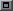=

 (dm = RWX scale)

Scale is referencing measurement of one thing against measurement of another thing. The relationship between the 2 things is scale. If the scale of an object is off, the object will look too big or small in relation to the surrounding objects. Keeping your objects' scales consistent will reduce disorientation and awkwardness while moving among them.

Conversion
RWX=AW=VRML
3D Studio (+Max)
trueSpace
.1m1m10m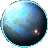Scene/World

In Active Worlds, zones and sectors divide the world up. I won't go into these since you probably won't need to worry about them and Roland does a good enough job of explaining them anyway. All we need to worry about are cells, which divide the world up even more.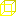Cell

Object

based off TAP's spec

Knowing how objects can be moved and manipulated within Active Worlds will help you design better objects that will be easier to build with. AW objects can be manipulated via keyboard and/or mouse (while it can be odd at first--and irritating after you right-click an object and accidentally move it--it can be helpful).

Keyboard
°: degree(s) symbol
%: percent symbol
½: 1/2, half, one-half; .5 ("point five")
¼: 1/4, fourth, one-forth; .25 ("point two-five" or "point twentyfive")

movement: one press of an arrow (left, right, up, down) or [+] (plus)/[-] (minus) key(s)
1 movement = .5m (50cm)
1 rotation = 15° (1/24, one-twenty-fourth of a circle)
3 rotations = 45° (1/8, one-eigth of a circle)
6 rotations = 90° (¼ circle)
12 rotations = 180° (½ circle)
24 rotations = 360° (full circle)

[Shift]: holding down the [Shift] key with either a movement or rotation
1 [Shift] movement = .05m (5cm, 10% of a movement)
1 [Shift] rotation = 1.5° (10% of a rotation)

[Ctrl]+[Shift]: holding down the [Ctrl] ("Control") key and (+) the [Shift] key with either a movement or rotation
1 [Control]+[Shift] movement = .01m (1cm, 1% of a movement)
1 [Control]+[Shift] rotation = .1° (1/150 or .006% of a rotation)

### Movement

movement[Shift][Ctrl]+[Shift]
1=10=50
1/10=1=5
1/50=1/5=1

### Rotation

rotation[Shift][Ctrl]+[Shift]
1=10=50
1/10=1=15
1/150=1/10=1

Note: keyboard controls may vary depending on how you have them configured.

^ Top